# The continuity of the function

## The continuity of the function at the point

Definition: a Functionis called continuous at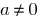if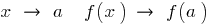, that is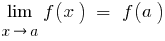.

## Continuous functions on the interval

Definition: If a function iscontinuous at each point of some interval, it is called continuous on the interval.

## Continuity properties of functions

1. If continuous on wtryskuindividual acquires at the ends of this segment the values of different signs, then at some point this segment she takes on a value of zero.
2. Example of continuity of a functionis a continuous function (polynomial)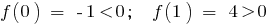so on the interval (0;1) there is a point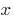where the function equals 0:3. If on the intervalthe function iscontinuous and does not turn into zero on this interval function keeps a constant sign.
4. Example of continuity of a function

Interval methods

5. A function, continuous on an intervaltakes on all intermediate values between the values of this function at the extreme points, i.e., between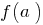and.
6. Example of continuity of a function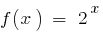is a continuous function. If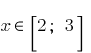then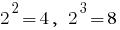. Because, there is a point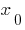where.7. A functioncontinuous on a segment, is restricted on this segment, then there exist two numbers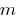andthat for all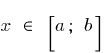inequality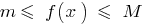.
8. The rule of finding the largest and smallest snakeb functions.

9. The amount of the difference and the work is continuous on this interval the function is continuous on the same interval function. The quotient of two continuous functions is a continuous function at all points where the denominator is not peretolchina to zero.
10. The inverse of a continuous function on the given interval, is continuous on this interval.
11. If the functionhas a derivative at, then it is continuous at that point.

## Break point

Definition: Point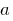break point function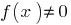, if the pointis not the condition that when.

### Examples of functions with break points— break points all the integer points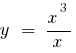— break - 0— break - 0

Tags:
Chapter:
Versions in other languages:
Share with friends: